Related Articles

# Synchronous Controlled Counter

• Difficulty Level : Expert
• Last Updated : 17 May, 2021

In this article, we will discuss the overview of the Synchronous controlled counter and will discuss its circuit diagram, circuit excitation table, timing diagram in detail. Let’s discuss it one by one.

Overview :
Toggle or D-type flip-flops can be used to make synchronous counters, and It is easy to design than asynchronous counters. Due to the clock input of flip-flops are all clocked together and with the same clock signal at the same time, that’s why it is called synchronous counters. It is also called parallel counters as the clock is fed in parallel to all flip-flops.

Prerequisite –
Design for the synchronous counter and the following pre-requisites as follows.

• In this type of counter mode control input is applied.
• Mode control input is decided which sequence is followed by a counter.
• The procedure for design is the same as synchronous counter designing.
• Any type of FF can be used for such a design.

Example :
Consider a counter that can count mod 4 or mod 8 is decided by mode control input (say S). If S= 0, then it will work as mod 4 counter, if S=1 it will perform down counting.

• For the designing of this type of counter 23= 8(because 3 bits are required for counting up to 7) i.e three FF are required. Here T FF is used. The state diagram is showing in the below picture.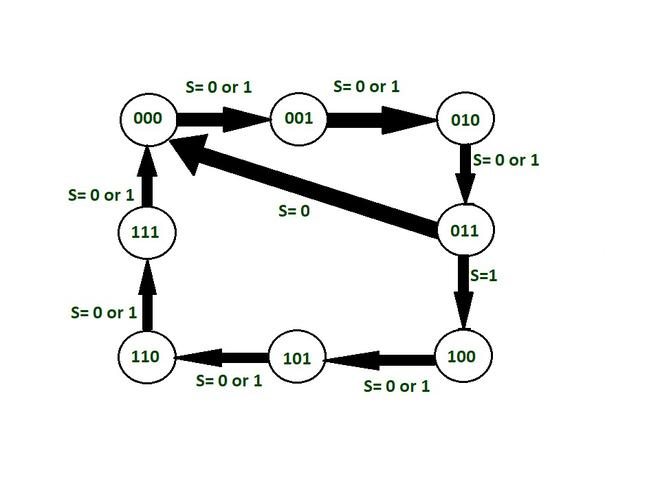• In this, if the counter state is below 011, the value of S can be 0 or 1 because in mod -4 or mod 8 the counting sequence up to 011 is same in both the situation is the same. So the value of S can be 0 or 1. The transition from 011 to 000 takes place if S= 0 and the counter will reset. The transition from 011 to 100 takes place if S= 1. After that, the value of S can be 0 or 1 until the state 000 arises.

Circuit excitation table :
It shows the present state of the FF and the next state after the clock pulse applied and the input value. Here T FF is used. So the value of T(toggle) input of FF is 1 only if the corresponding state output value changed from 0 to 1 or 1 to 0. Otherwise, it remains the same. The below table is according to the required counting sequence.

```Q  - means Present state
Q* - means next state.```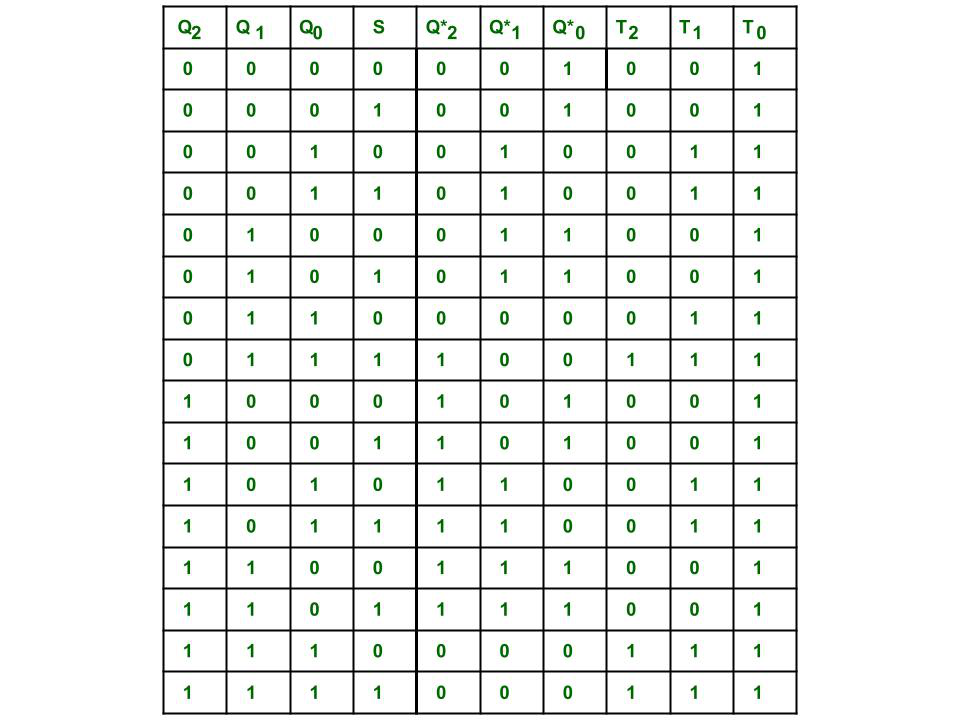Here we are finding out the minimal boolean expression for input variable for 3 Flip flops using K map.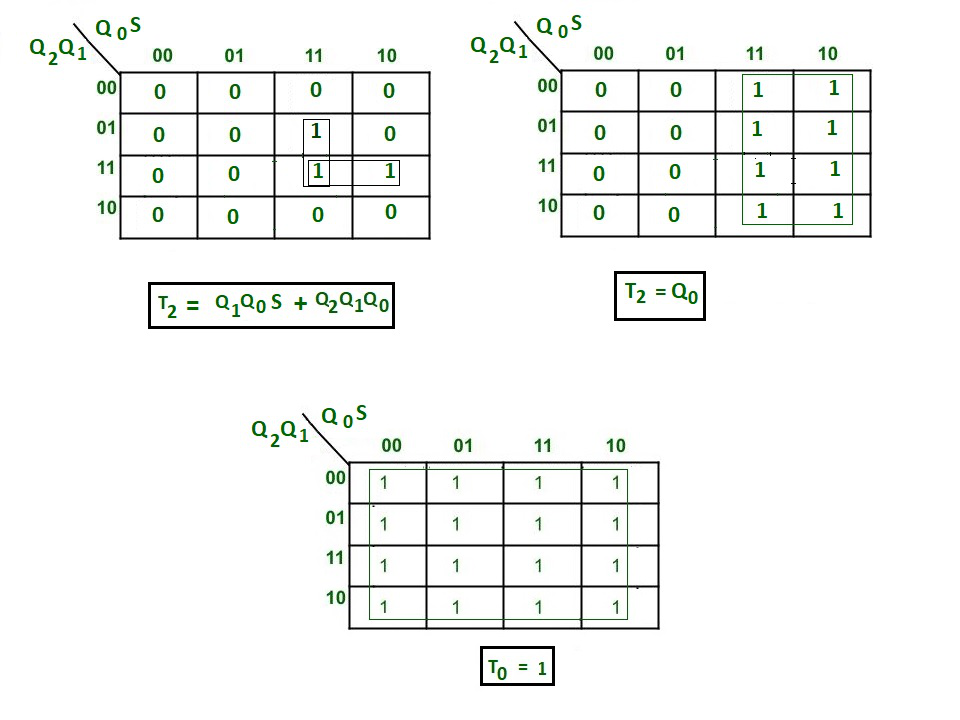Circuit Diagram :
Here the circuit is implemented for expressions that we get already. The circuit output sequence is the same as shown in the state diagram.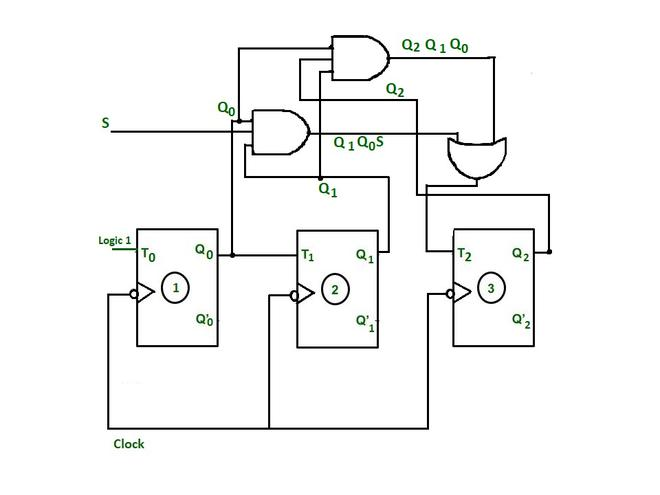Timing diagram :
The sequence of the counters can be verified from the timing diagram. If the counter is reset and the control input S is 0 then it will follow the sequence of mod– 4 i.e. it will count 000, 001, 010, 011 and repeats. If if the counter is reset and the control input S is 1, then the counter will count the sequence 000, 001, 010, 011, 100, 101, 110, 111, and repeats.

Case-1
When S= 0, after 4th -ve edge triggered clock pulse.

• When Q2=0, Q1= 1, Q0 = 1 and S=0, i.e state of 3 FF after 3rd -ve edge clock pulse.
• Put these into expression T2 = Q2Q1Q0 + SQ1Q0= 0+0 =0, then after 4th -ve edge triggered clock pulse FF 3 reserve its state i.e. Q2 = 0
• T1= Q0 = 1, therefore FF 2 toggles its output state ie Q1 becomes 0
• T0 = 1, therefore FF 1 toggle its output state i.e. Q0 becomes 0
• Q2 Q1 Q0 becomes after 4th negative clock 0 0 0 whose decimal equivalent is 0
• So the transition 110 to 000 (reset) takes place after 4th -Ve edge.

Case-2
When S=1, after 4th -ve edge-triggered clock pulse.

• When Q2=0, Q1= 1, Q0 = 1 and S=1, i.e state of 3 FF after 3rd -ve edge clock pulse.
• Put these into expression T2 = Q2Q1Q0 + SQ1Q0= 0+1= 1, then at 4th -ve edge clock pulse FF 3 reserve its toggles its output state i.e. Q2 becomes 1.
• T1= Q0 = 1,therefore FF 2 toggles its output state ie Q1 becomes 0
• T0 = 1, therefore FF 1 toggle its output state i.e. Q0 becomes 0
• Q2 Q1 Q0 becomes at 4th negative clock 1 0 0 whose decimal equivalent is 4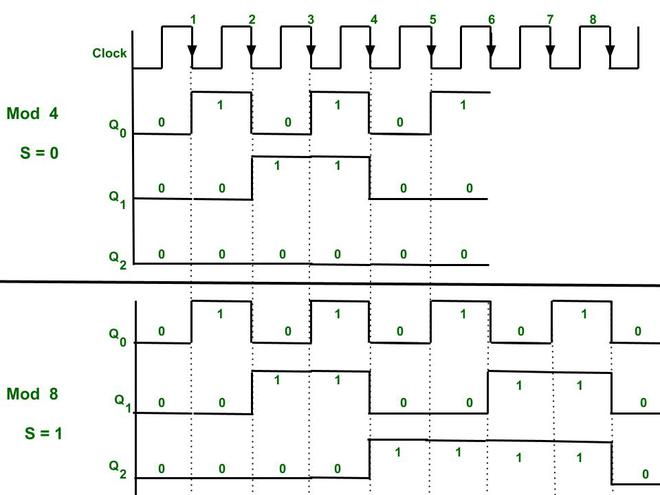The timing diagram for mod-4 or mod-8 counter

Explanation
Here the -Ve edge triggering is used for toggling purposes.

• As T1= Q0. Therefore, the output state of FF 1 will change only when there is a falling edge(i.e -ve edge-triggered clock is used) and Q0 =1, then toggling occur and the output state of T1 will change.
• As T2 = Q1Q0S+ Q2Q1Q0. If S= 0 then the output state of FF 2 will change only when Q2Q1Q0 will result in 1 and also there is a falling edge otherwise the state is preserved.
• If S= 1, T2 = Q1Q0+ Q2Q1Q0, therefore output state of FF 3 will change when Q1Q0 result is 1 or Q2Q1Q0 results 1 and there is clock falling edge.
• Therefore, at every -Ve edge clock pulse the output state changes.

Attention reader! Don’t stop learning now.  Practice GATE exam well before the actual exam with the subject-wise and overall quizzes available in GATE Test Series Course.

Learn all GATE CS concepts with Free Live Classes on our youtube channel.

My Personal Notes arrow_drop_up